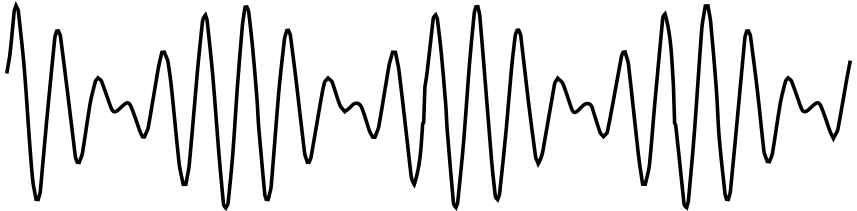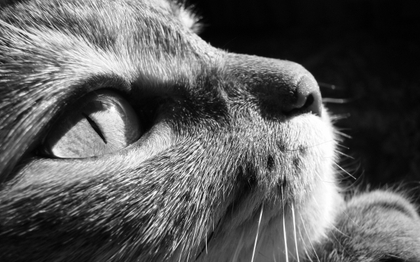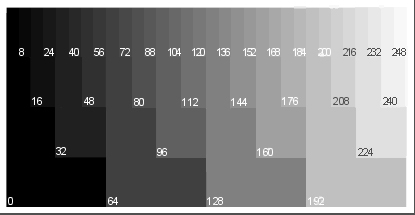## Welcome back

### to “Computing for Molecular Biology 1”

• why computing is important to us
• what is a computer
• what a computer can do
• some parts of a computer
• some strategies for effective learning

## The smallest information piece

The most simple answer to a question is yes or no

When we bet on a tossed coin, what do we know?

This elementary information unit is called bit
(binary digit)

It can be represented by on/off, true/false, 0/1, etc.

## Binary representation

For technical reasons modern computers handle only packs of 8 bits

That is called a byte and can represent a number in the range 0 to 255

Using two bytes we can represent numbers between 0 and 65535

How? If $$x$$ and $$y$$ are two bytes, we can evaluate $x+256 y$

## Bigger numbers

### binary representation

The idea can be extended to using 4 bytes

0 to 4 294 967 295

and also to using 8 bytes

From 0 to 18 446 744 073 709 551 615 $1.8466 \cdot 10^{19}$

## Signed numbers

With a small modification we can also represent negative numbers.

For example, a number $$x$$ between -128 and 127 can be represented as $$x+128$$

Note: in practice we use a similar but different encoding

## Example: Sound

• Sound is transformed into electricity by a microphone.
• The voltage is measured 44100 times each second
• Each sample is stored as a number in a CD

Two steps: sampling (in time) and discretization (in voltage)## Example: Greyscale Imagecats

## Example: Greyscale Image

• Each “point” has a value between 0 (black) and 255 (white)
• correct name is pixel picture element
• they are stored line by linegreyscale

## Floating point

Using scientific notation we write $1.8466 \cdot 10^{19}$ Using the same idea we can use two numbers like this $$x\cdot 2^y$$

There are two versions: single and double precision

They use 4 and 8 bytes, respectively

## Floating point standard

Notice that this approach has some limitations

Not all numbers are represented exactly

Can also represent special values

• Inf: Positive Infinity, 1/0
• -Inf: Negative Infinity, -1/0
• NaN: Not a number, 0/0
• NA: Not Available, missing data

## Two kinds of memory

The computer has memory to store information. These are electronic devices

When the computer is turned off, the information is lost

We need to copy information to a secondary storage

## Secondary storage examples

• hard disk
• flash disk (USB stick)
• cloud storage
• diskette/floppy disk
• zip disk
• tape
• punched cards

## Memory size

How much can we store in the computer?

What is the size of the memory of your computer?

What is the size of the disk?

The memory (RAM) is like a desk.
The disk is like a book shelf.

## Representing text

The most natural way to represent a text document is to encode each letter with a single byte

There is a basic standard for English, called ASCII

Each number from 0 to 127 is either a symbol or a special signal, such as

• New Line
• End of Message
• Tab
• Space
• Backspace

## ASCII code

30 40 50 60 70 80 90 100 110 120
0 ( 2 < F P Z d n x
1 ) 3 = G Q $| e | o | y 2| | | 4| >| H| R|\\| f | p | z 3| !| +| 5| ?| I| S|$ g q {
4 " , 6 @ J T ^ h r |
5 # - 7 A K U i s }
6 \$ . 8 B L V  j t ~
7 % / 9 C M W a k u
8 & 0 : D N X b l v
9 ´ 1 ; E O Y c m w

Numbers between 128 and 255 are not used in ASCII

Non English languages use these values for symbols like “Ç”, “Ö”, “É”, “Ñ”

## Text Files

• are universal
• are easy to read and write from a program
• do not have any style like bold or italic
• are like books without figures

Microsoft Word files (doc or docx`) are NOT text files

Thou shall not use Word for this course